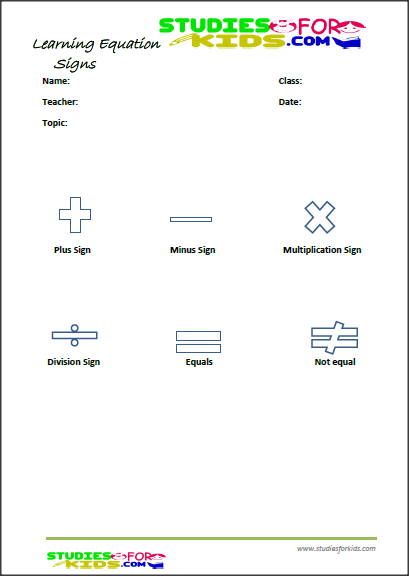# Free printable Equation signs activities for kids learning Pdf

PDF:basic Equation signs activities for kids.pdfFree printable Equation signs activities for kids learning pdf is the main learning activities for kids on this learning page. This page consists of basic equation signs such as plus sign, minus sign, multiplication sign, division sign, equal sign, and not equal sign as well for kids. In this activities for kids, kids will be able to learn about all the equation signs by identifying them with the help of their tutors or parents. Considering the size of the images, kids will be able to retain the mathematical signs such as the equal sign as they are being thought. This Free Printable Equation signs activities for kids learning has been proven to be helpful for kids learning. Click on the PDF link or image to download this free printable learning worksheet activities for kids as they learn about the equation signs.